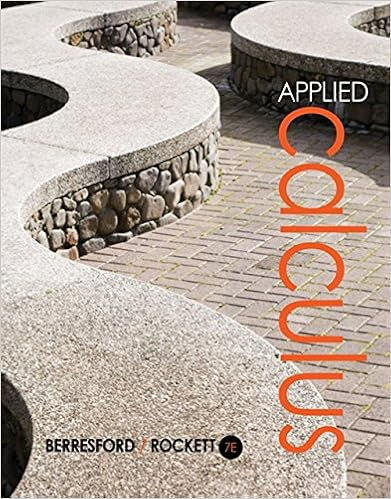# A monopolistically competitive firm maximizing

• Notes
• 15
• 96% (24) 23 out of 24 people found this document helpful

This preview shows page 6 - 8 out of 15 pages.

##### We have textbook solutions for you!
The document you are viewing contains questions related to this textbook.The document you are viewing contains questions related to this textbook.
Chapter 3 / Exercise 62
Applied Calculus
Berresford/RockettExpert Verified
27) A monopolistically competitive firm maximizing profits will produce at a price that is: A) equal to marginal revenue. B) greater than marginal cost. C) equal to marginal cost. D) less than marginal revenue. Answer:
B Diff: 2 Page Ref: 394 28) Which of the following is NOT a characteristic of long-run equilibrium in a monopolistically competitive market?
A Diff: 2 Page Ref: 401 29) A monopolistically competitive industry that earns economic profits in the short run will:
C Diff: 2 Page Ref: 396 30) Monopolistic competition has:
A Diff: 1 Page Ref: 390 31) Most gasoline stations usually have unused gasoline pumps which is evidence of: A) charging too high a price. B) excess capacity. C) a lack of advertising. D) marginal costs greater than marginal revenue. Answer:
B Diff: 2 Page Ref: 401 32) If a monopolistically competitive firm is producing 50 units of output where marginal cost equals marginal revenue, total cost is \$1,674 and total revenue is \$2,000, its average profit is:
##### We have textbook solutions for you!
The document you are viewing contains questions related to this textbook.The document you are viewing contains questions related to this textbook.
Chapter 3 / Exercise 62
Applied Calculus
Berresford/RockettExpert Verified
B Diff: 2 Page Ref: 393 Refer to Figure 12.1 for the questions below.Figure 12.1 33) The productive efficient output for the monopolistic competitor in figure 12.1 is:
D Diff: 3 Page Ref: 396 34) A major difference between monopolistically competitive and perfectly competitive markets is: A) the degree by which market demand slopes downward. B) the number of sellers. C) barriers to entry. D) products are not standardized in monopolistic competition. Answer:
D Diff: 2 Page Ref: 401 35) A franchise is:
C Diff: 2 Page Ref: 400
•••